# The Go image package

## Introduction

The image and image/color packages define a number of types: `color.Color` and `color.Model` describe colors, `image.Point` and `image.Rectangle` describe basic 2-D geometry, and `image.Image` brings the two concepts together to represent a rectangular grid of colors. A separate article covers image composition with the image/draw package.

## Colors and Color Models

Color is an interface that defines the minimal method set of any type that can be considered a color: one that can be converted to red, green, blue and alpha values. The conversion may be lossy, such as converting from CMYK or YCbCr color spaces.

``````type Color interface {
// RGBA returns the alpha-premultiplied red, green, blue and alpha values
// for the color. Each value ranges within [0, 0xFFFF], but is represented
// by a uint32 so that multiplying by a blend factor up to 0xFFFF will not
// overflow.
RGBA() (r, g, b, a uint32)
}
``````

There are three important subtleties about the return values. First, the red, green and blue are alpha-premultiplied: a fully saturated red that is also 25% transparent is represented by RGBA returning a 75% r. Second, the channels have a 16-bit effective range: 100% red is represented by RGBA returning an r of 65535, not 255, so that converting from CMYK or YCbCr is not as lossy. Third, the type returned is `uint32`, even though the maximum value is 65535, to guarantee that multiplying two values together won’t overflow. Such multiplications occur when blending two colors according to an alpha mask from a third color, in the style of Porter and Duff’s classic algebra:

``````dstr, dstg, dstb, dsta := dst.RGBA()
srcr, srcg, srcb, srca := src.RGBA()
_, _, _, m := mask.RGBA()
const M = 1<<16 - 1
// The resultant red value is a blend of dstr and srcr, and ranges in [0, M].
// The calculation for green, blue and alpha is similar.
dstr = (dstr*(M-m) + srcr*m) / M
``````

The last line of that code snippet would have been more complicated if we worked with non-alpha-premultiplied colors, which is why `Color` uses alpha-premultiplied values.

The image/color package also defines a number of concrete types that implement the `Color` interface. For example, `RGBA` is a struct that represents the classic “8 bits per channel” color.

``````type RGBA struct {
R, G, B, A uint8
}
``````

Note that the `R` field of an `RGBA` is an 8-bit alpha-premultiplied color in the range [0, 255]. `RGBA` satisfies the `Color` interface by multiplying that value by 0x101 to generate a 16-bit alpha-premultiplied color in the range [0, 65535]. Similarly, the `NRGBA` struct type represents an 8-bit non-alpha-premultiplied color, as used by the PNG image format. When manipulating an `NRGBA`’s fields directly, the values are non-alpha-premultiplied, but when calling the `RGBA` method, the return values are alpha-premultiplied.

A `Model` is simply something that can convert `Color`s to other `Color`s, possibly lossily. For example, the `GrayModel` can convert any `Color` to a desaturated `Gray`. A `Palette` can convert any `Color` to one from a limited palette.

``````type Model interface {
Convert(c Color) Color
}

type Palette []Color
``````

## Points and Rectangles

A `Point` is an (x, y) co-ordinate on the integer grid, with axes increasing right and down. It is neither a pixel nor a grid square. A `Point` has no intrinsic width, height or color, but the visualizations below use a small colored square.

``````type Point struct {
X, Y int
}
``````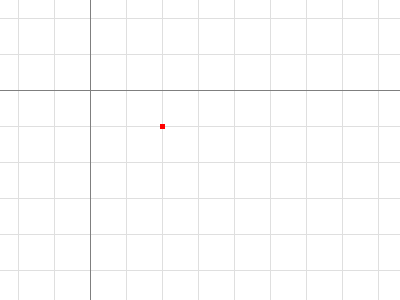``````p := image.Point{2, 1}
``````

A `Rectangle` is an axis-aligned rectangle on the integer grid, defined by its top-left and bottom-right `Point`. A `Rectangle` also has no intrinsic color, but the visualizations below outline rectangles with a thin colored line, and call out their `Min` and `Max` `Point`s.

``````type Rectangle struct {
Min, Max Point
}
``````

For convenience, `image.Rect(x0, y0, x1, y1)` is equivalent to `image.Rectangle{image.Point{x0, y0}, image.Point{x1, y1}}`, but is much easier to type.

A `Rectangle` is inclusive at the top-left and exclusive at the bottom-right. For a `Point p` and a `Rectangle r`, `p.In(r)` if and only if `r.Min.X <= p.X && p.X < r.Max.X`, and similarly for `Y`. This is analogous to how a slice `s[i0:i1]` is inclusive at the low end and exclusive at the high end. (Unlike arrays and slices, a `Rectangle` often has a non-zero origin.)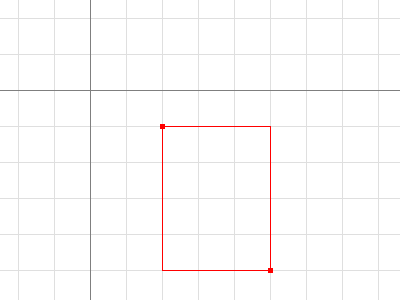``````r := image.Rect(2, 1, 5, 5)
// Dx and Dy return a rectangle's width and height.
fmt.Println(r.Dx(), r.Dy(), image.Pt(0, 0).In(r)) // prints 3 4 false
``````

Adding a `Point` to a `Rectangle` translates the `Rectangle`. Points and Rectangles are not restricted to be in the bottom-right quadrant.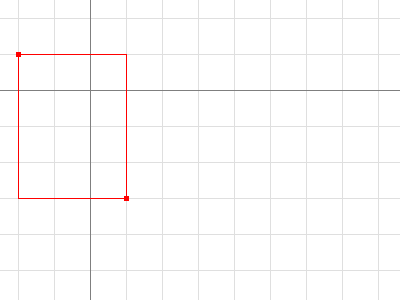``````r := image.Rect(2, 1, 5, 5).Add(image.Pt(-4, -2))
fmt.Println(r.Dx(), r.Dy(), image.Pt(0, 0).In(r)) // prints 3 4 true
``````

Intersecting two Rectangles yields another Rectangle, which may be empty.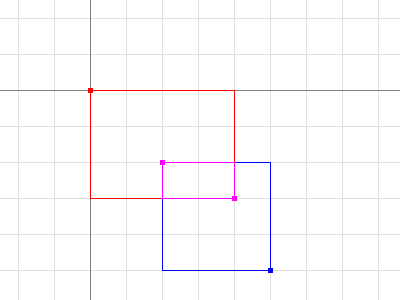``````r := image.Rect(0, 0, 4, 3).Intersect(image.Rect(2, 2, 5, 5))
// Size returns a rectangle's width and height, as a Point.
fmt.Printf("%#v\n", r.Size()) // prints image.Point{X:2, Y:1}
``````

Points and Rectangles are passed and returned by value. A function that takes a `Rectangle` argument will be as efficient as a function that takes two `Point` arguments, or four `int` arguments.

## Images

An Image maps every grid square in a `Rectangle` to a `Color` from a `Model`. “The pixel at (x, y)” refers to the color of the grid square defined by the points (x, y), (x+1, y), (x+1, y+1) and (x, y+1).

``````type Image interface {
// ColorModel returns the Image's color model.
ColorModel() color.Model
// Bounds returns the domain for which At can return non-zero color.
// The bounds do not necessarily contain the point (0, 0).
Bounds() Rectangle
// At returns the color of the pixel at (x, y).
// At(Bounds().Min.X, Bounds().Min.Y) returns the upper-left pixel of the grid.
// At(Bounds().Max.X-1, Bounds().Max.Y-1) returns the lower-right one.
At(x, y int) color.Color
}
``````

A common mistake is assuming that an `Image`’s bounds start at (0, 0). For example, an animated GIF contains a sequence of Images, and each `Image` after the first typically only holds pixel data for the area that changed, and that area doesn’t necessarily start at (0, 0). The correct way to iterate over an `Image` m’s pixels looks like:

``````b := m.Bounds()
for y := b.Min.Y; y < b.Max.Y; y++ {
for x := b.Min.X; x < b.Max.X; x++ {
doStuffWith(m.At(x, y))
}
}
``````

`Image` implementations do not have to be based on an in-memory slice of pixel data. For example, a `Uniform` is an `Image` of enormous bounds and uniform color, whose in-memory representation is simply that color.

``````type Uniform struct {
C color.Color
}
``````

Typically, though, programs will want an image based on a slice. Struct types like `RGBA` and `Gray` (which other packages refer to as `image.RGBA` and `image.Gray`) hold slices of pixel data and implement the `Image` interface.

``````type RGBA struct {
// Pix holds the image's pixels, in R, G, B, A order. The pixel at
// (x, y) starts at Pix[(y-Rect.Min.Y)*Stride + (x-Rect.Min.X)*4].
Pix []uint8
// Stride is the Pix stride (in bytes) between vertically adjacent pixels.
Stride int
// Rect is the image's bounds.
Rect Rectangle
}
``````

These types also provide a `Set(x, y int, c color.Color)` method that allows modifying the image one pixel at a time.

``````m := image.NewRGBA(image.Rect(0, 0, 640, 480))
m.Set(5, 5, color.RGBA{255, 0, 0, 255})
``````

If you’re reading or writing a lot of pixel data, it can be more efficient, but more complicated, to access these struct type’s `Pix` field directly.

The slice-based `Image` implementations also provide a `SubImage` method, which returns an `Image` backed by the same array. Modifying the pixels of a sub-image will affect the pixels of the original image, analogous to how modifying the contents of a sub-slice `s[i0:i1]` will affect the contents of the original slice `s`.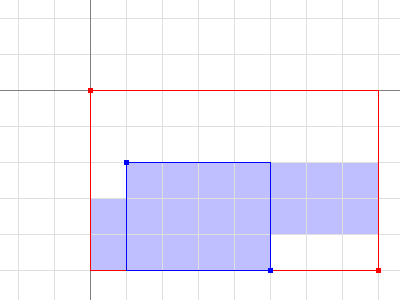``````m0 := image.NewRGBA(image.Rect(0, 0, 8, 5))
m1 := m0.SubImage(image.Rect(1, 2, 5, 5)).(*image.RGBA)
fmt.Println(m0.Bounds().Dx(), m1.Bounds().Dx()) // prints 8, 4
fmt.Println(m0.Stride == m1.Stride)             // prints true
``````

For low-level code that works on an image’s `Pix` field, be aware that ranging over `Pix` can affect pixels outside an image’s bounds. In the example above, the pixels covered by `m1.Pix` are shaded in blue. Higher-level code, such as the `At` and `Set` methods or the image/draw package, will clip their operations to the image’s bounds.

## Image Formats

The standard package library supports a number of common image formats, such as GIF, JPEG and PNG. If you know the format of a source image file, you can decode from an `io.Reader` directly.

``````import (
"image/jpeg"
"image/png"
"io"
)

// convertJPEGToPNG converts from JPEG to PNG.
func convertJPEGToPNG(w io.Writer, r io.Reader) error {
img, err := jpeg.Decode(r)
if err != nil {
return err
}
return png.Encode(w, img)
}
``````

If you have image data of unknown format, the `image.Decode` function can detect the format. The set of recognized formats is constructed at run time and is not limited to those in the standard package library. An image format package typically registers its format in an init function, and the main package will “underscore import” such a package solely for the side effect of format registration.

``````import (
"image"
"image/png"
"io"

_ "image/jpeg"
)

// convertToPNG converts from any recognized format to PNG.
func convertToPNG(w io.Writer, r io.Reader) error {
img, _, err := image.Decode(r)
if err != nil {
return err
}
return png.Encode(w, img)
}
``````

Next article: The Go image/draw package
Previous article: The Laws of Reflection